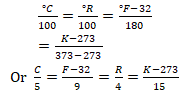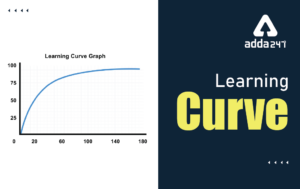# Heat And Temperature: – Download Science Study Notes Free PDF For REET/UTET ExamIn many teaching exams including  REETUTET DSSSB Exams etc. Science may be an interesting subject having and 30 questions of Science content and 30 questions of Science Pedagogy in REETUTET  and other State TET Exams. Science comprising of various branches of studies like chemistry, physical science, and life science.

Get free Study material for REET Exam

## HEAT AND TEMPERATURE

• The energy transferred from one body to another due to temperature difference between them is called heat.
• The quantity by which you can compare how hot or cold a body is called temperature.
• In CGS system, the unit of heat is calorie. It is equal to the quantity of heat required to raise the temperature of heat one gram of water through 1°C.
• C° (Celsius), °F (Fahrenheit) and K (Kelvin) are the units of measuring temperature.

1. Celsius scale

• On this scale, ice point is 0°C and steam point is 100°C. The distance between these two fixed points is divided into 100 equal parts. Each part corresponds to a difference to temperature of 1°C.

2. Kelvin Scale

• On this scale, ice point is 273 K and steam point 373K. The distance between these two fixed points is divided into 180 equal parts. Each part corresponds to a difference of temperature of 1 K.

3. Specific heat

• The specific heat of a substance is the amount of heat, which is required to raise the temperature of a unit mass of the substance by 1°C. Its SI unit is Cal/g °C or J/kg °C.

4. Thermal Capacity

• The thermal capacity of a body is the amount of heat, which is required to raise the temperature of a unit mass of the substance by 1°C. Its SI unit is Cal/g °C or J/kg °C.

5. Melting Point:

• The temperature at which a substance changes its state from solid to liquid is called its melting point.

6. Boiling Point:

• The temperature at which a substance changes its state liquid to gas is called its boiling point.
• The Si unit of heat is joule (J).

7. Calorie:

• The calorie is defined as the amount, of heat required to raise the temperature of 1g of water by 1°C.

1Cal = 4.184J

1 Kilo Cal = 1000cal

How To Score 25+ in Science For TET Exam

Heat and Capacity:

• The heat capacity of a given amount of a substance is the amount of heat required to raise temperature by 1°C. Its SI unit is joule/K (J/K)
• Amount of heat = Mass × Specific heat capacity × Rise in temperature

e.g. Thus, when the temperature of 1kg of water is increased by 1°C, the heat required is 41; therefore, the amount of heat (Q) supplied to raise the temperature of 10 kg of water by 10°C  will be

Q = (10 kg) × (4186 J/kg°C) ×(10°C)

= 4.186 × 10⁵J

• Amount of heat lost = Mass × Specific heat × Decrease in temperature

General,

Heat gained or lost = Mass × Specific heat capacity × Change in temperature

Transfer of Heat:

• There are three way to transmission of heat
1. Conduction
2. Convection

GET SCIENCE STUDY NOTES HERE

Conduction:

It is a process through which heat is transfer in solids.

• Transfer of energy between different parts of a body or from one body to another in contact with it, is called conduction.
• Important condition for conduction
• The two object should be in contact and
• Their temperature should be different.

Convection:

The process of the hotter fluid moving and transferring heat to the colder surrounding is called convection.

Example: a. Sea breeze during the daytime, the warm air from the land move towards the sea and cooler air from the sea rushes in towards the land to take it place. The air from the sea is called the sea breeze.

b. At night, the cool air from the land moves towards the sea, this is called the land breeze.

It is a process by which heat travels without the help of a material medium, black objects are the best radiation of heat.

Conductor: Substance, through which heat flows easily, is called conductor.

• Metals are good conductors, silver is the best conductor.
• Woolen clothes keeps us warm during winter. It is so because wool is a poor conductor of heat and it has air trapped in between the fibers.
• The range of laboratory thermometer is generally from – 10°C to 110°C.
• The thermometer that measures our body temperature is called a clinical thermometer.

Insulator: Substances, through which heat does not flow easily, are called insulator.

• Paper, glass, wood, plastic are insulator.
• Air is bas conductor of heat.

GET COMPLETE EVS STUDY NOTES HERE

Thermometer: It is a device, which is used for measurement of temperature.

Reaumur scale: In this scale lower fixed point is 0°R and upper fixed point is 80°R. The scale is divided into 80 divisions of all the equal size.

• Relation between different scalesOr

where C, → Celsius scale, R→ Reaumur scale, F→ Fahrenheit scale and K→ Kelvin scale.

• Normal temperature of human body is 37°C or 98.6°F.
• At – 40°C, the Celsius and Fahrenheit scales coincide.

Absolute Humidity: The mass of water vapor present in one cubic metre of atmospheric air is called absolute humidity. It is expressed in g/m³.

Dew point: The temperature at which the water vapor in a given volume becomes saturated in called dew point.

• Latent heat is defined as the energy required by unit mass of substance to change from one state of another without any variation in temperature.
• Latent heat of fusion of ice is 80 Cal/g or 335 J/g.
• Latent heat of vaporization of water is 540 Cal/g or 2260 J/g.

Practice REET Previous Year EVS Quiz For REET Exam Here

 Melting Point of Some Substances Solid Melting Point Ice 0°C Iron 1535°C Zinc 419.58°C Wax 63°C
 Boiling Point of Some Substances Substance Boiling point Water 100°C Mercury 356.58°C Copper 2336°C Zinc 907°C

•Learning Curve - What is Learning Curve?...
•रस - परिभाषा, भे�...
•पठन कौशल- परिभा�...
•अलंकार - परिभाष�...
•Indian States and Capitals 2022: 28 Stat...
•New Education Policy(NEP) PDF - नई �...